11.5 Confinement of positive ions and electrons with a static electric and magnetic field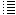Visualizing the electric field and some experiments

In the simulation program we can now visualize the electric field in the plane x= 0,5 mtr; see the images below.

The direction of the electric field is indicated by the arrows (this corresponds to the direction of the force a positive particle would experience).
The strength of the electric field is indicated by the color: the brighter red, the stronger the field.

Fig. 1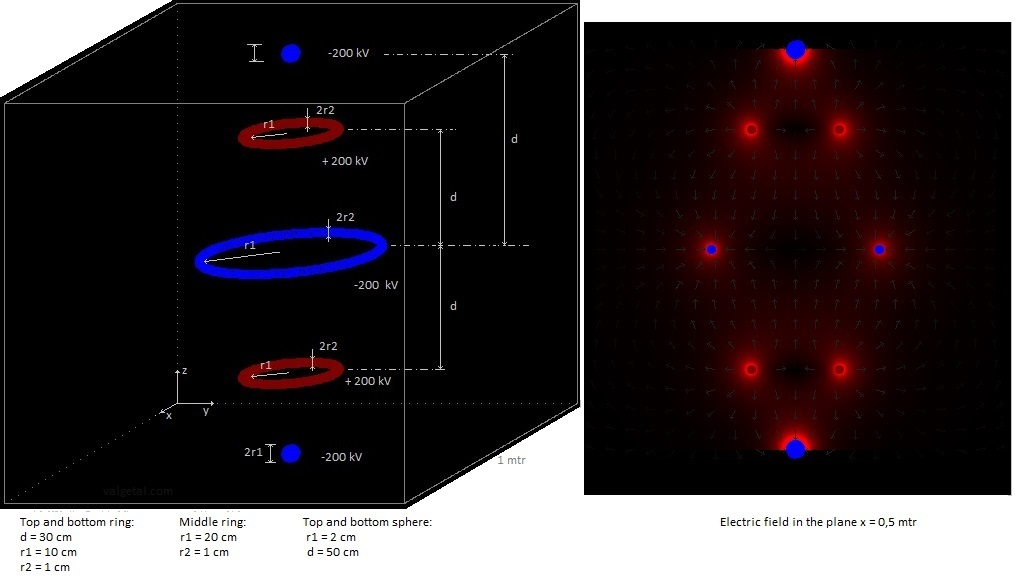Fig. 2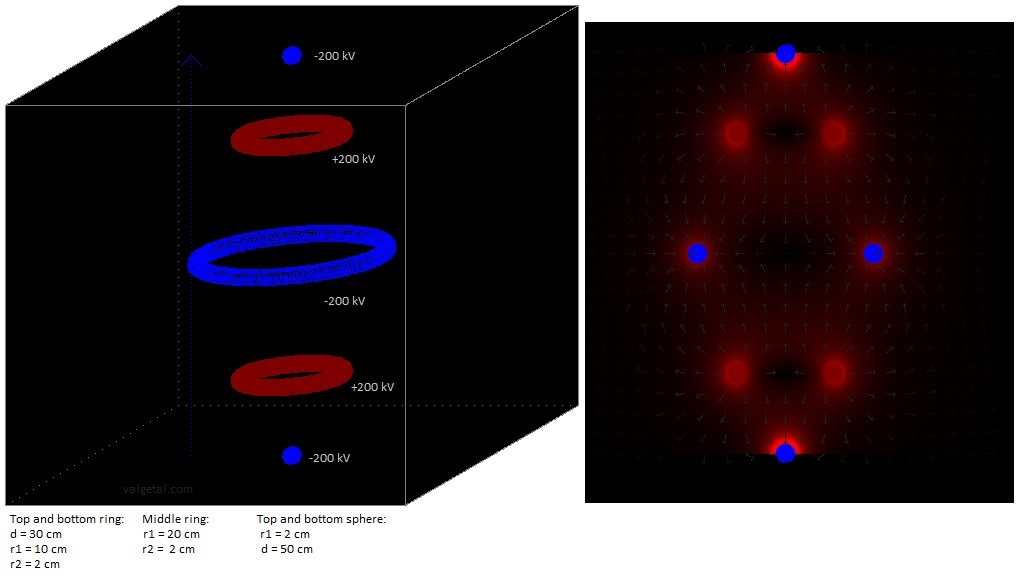Fig. 3.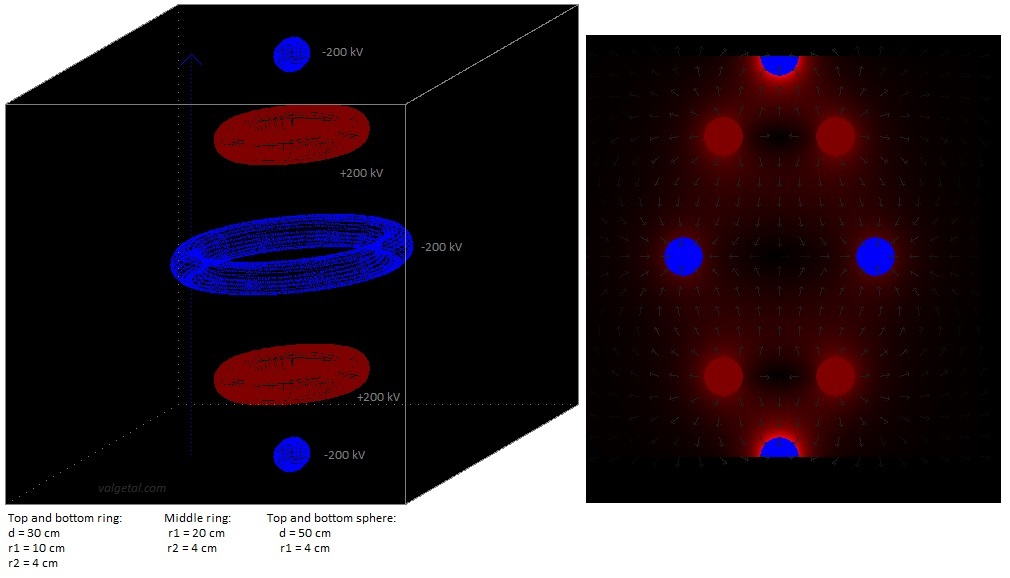Fig. 4.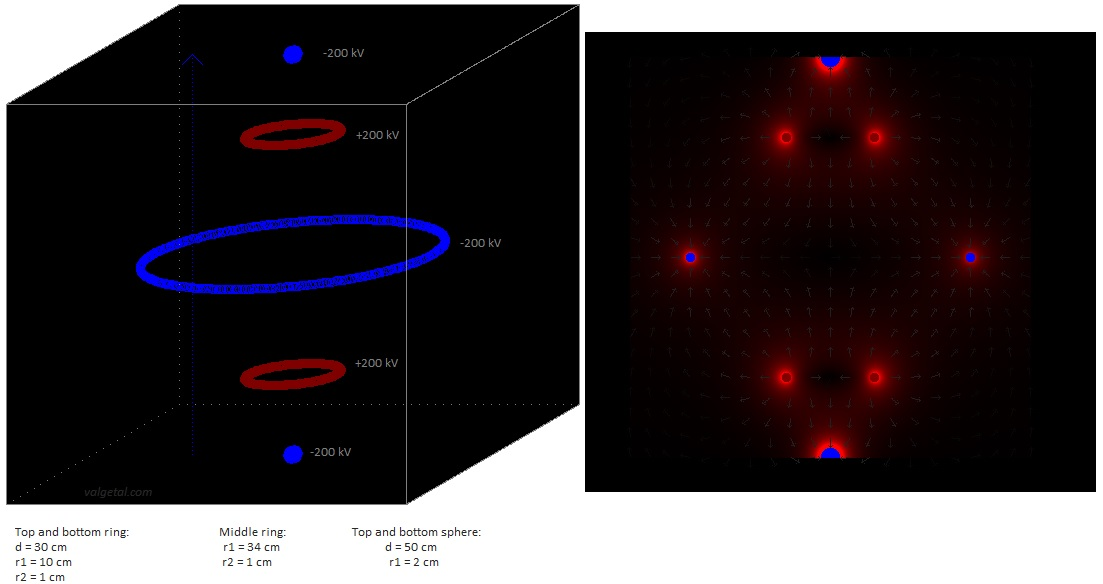Fig. 5.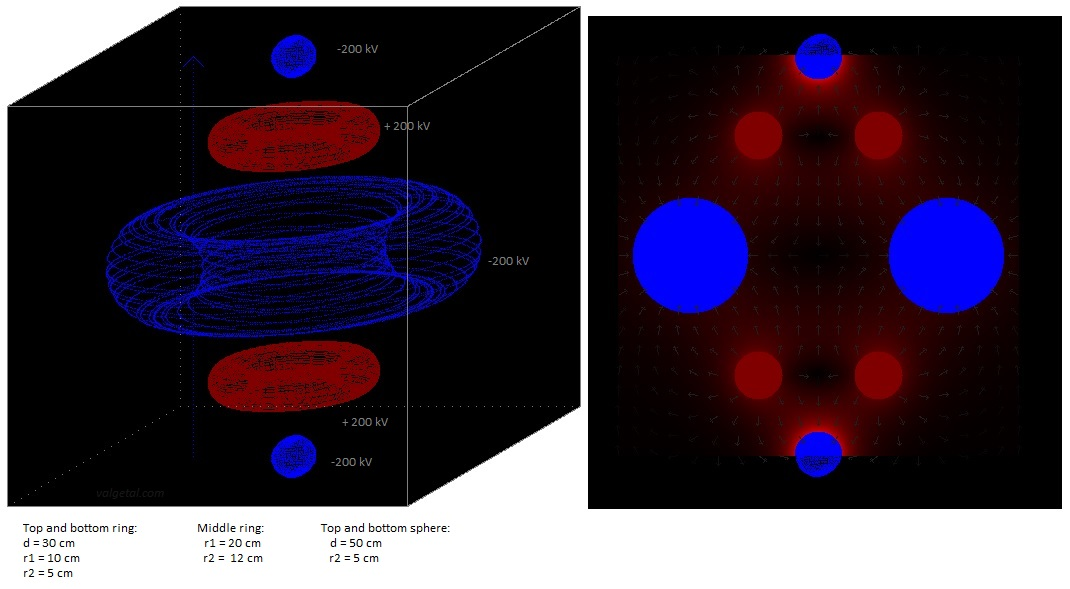Fig. 6a. Experiment with B = 2 T and the smallest possible size & fig.6b Potential (red = +,  blue = - )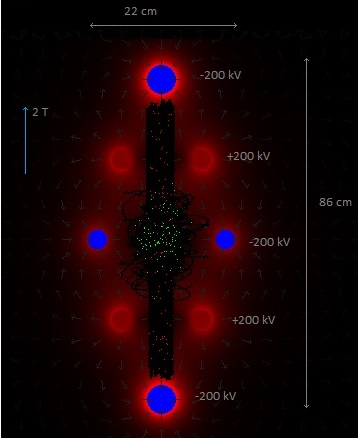Fig. 7. Experiment with B = 1.5 T  & fig.7b Potential (red = +,  blue = - )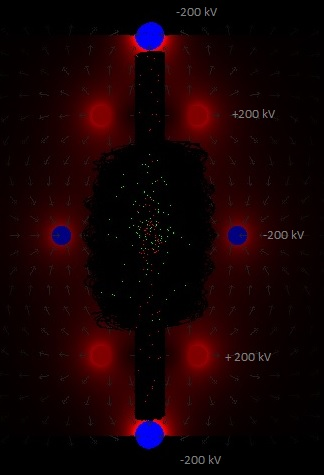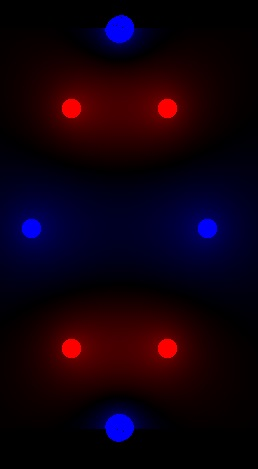Fig. 8a.. Experiment with B = 1.5 T  & & fig.8b. Potential (red = +,  blue = - )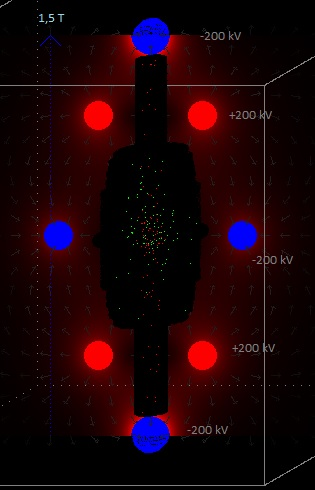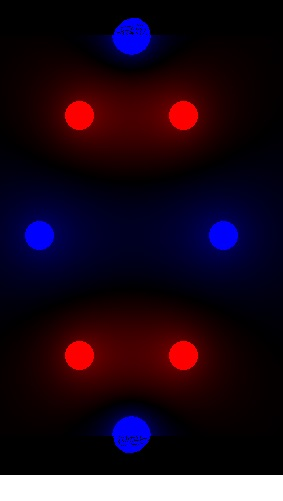The rings have a greater diameter. With the same voltage, they produce a stronger electric field
and the particles will be more confined. This can be seen comparing figure 7, 8 and 9.

Fig. 9a. Experiment with B = 1.5 T  & fig.9b Potential (red = +,  blue = - )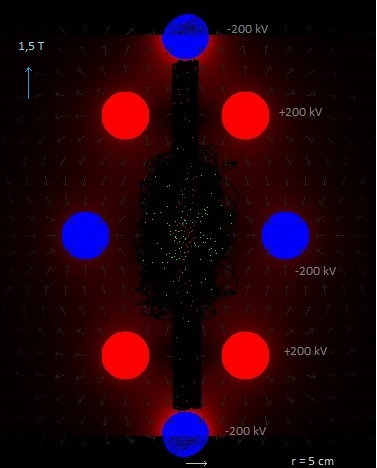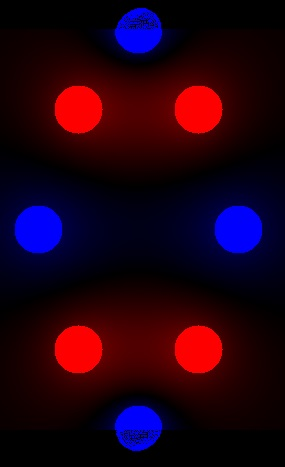Fig. 10a. Experiment with B = 1.5 T  &  fig.10b Potential (red = +,  blue = - )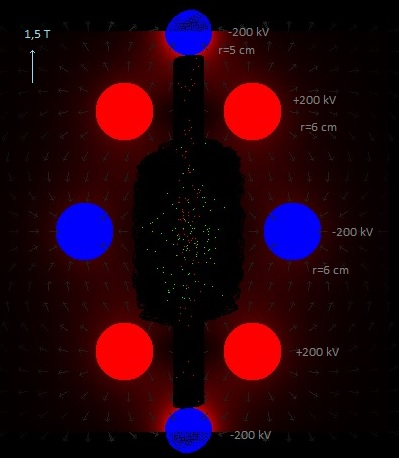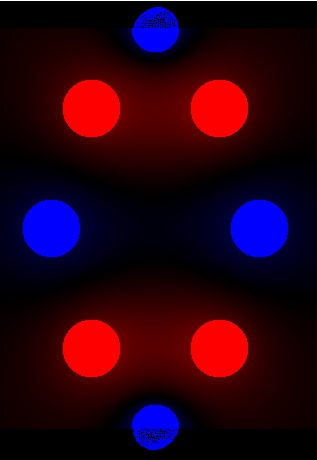17 May 2017 ..     by  Rinze Joustra        www.valgetal.com